# Civil Engineering - Water Supply Engineering

### Exercise :: Water Supply Engineering - Section 2

46.

A water channel supported above the ground over trestles, is generally called

 A. flume B. canal C. adueduct D. tunnel E. all the above.

Explanation:

No answer description available for this question. Let us discuss.

47.

If d is the diameter of the pipe, p is the total internal pressure, f is the permissible tensile stress and n is the effective of the joint, the thickness t of metal pipe, is

 A.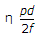B.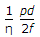C.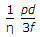D.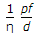E. none of these.

Explanation:

No answer description available for this question. Let us discuss.

48.

One degree of hardness of water means a content of salts of

 A. 10.25 mg/litre B. 12.25 mg/litre C. 14.25 mg/litre D. 16.25 mg/litre. E. 20 mg/litre.

Explanation:

No answer description available for this question. Let us discuss.

49.

Mostly used coagulant, is

 A. chlorine B. alum C. lime D. bleaching powder.

Explanation:

No answer description available for this question. Let us discuss.

50.

If G is the specific gravity of particles of diameter d, the velocity of settlement V in still water at T°C, according to Stoke's law, is

 A.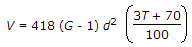B.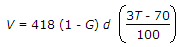C.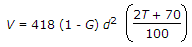D.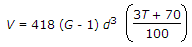E. none of these.